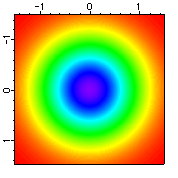Igor provides an extensive library of math and data manipulation routines and Igor's array-oriented arithmetic make complex operations a snap.

Igor provides all the mathematical operators and functions you would expect -- and then some. You can quickly find the desired function or operation using Igor's handy help browser as illustrated to the right.

Many of Igor's algorithms are from Numerical Recipes and the LAPACK numerical library.

Array arithmetic is the most flexible and powerful part of Igor's analysis capability. It allows you to write assignment statements that work on an entire Array or on a subset of an Array much as you would write an assignment to a single variable in a standard programming language.

You can access all of the most common operations via easy-to-use dialogs. Later, as you learn from watching as the dialogs synthesize commands, you can type directly on Igor's command line or write routines to perform specialized operations.By way of example, here are the command lines that create the data and the image plot shown on the right:

```Make/N=(150,150) data1     // create a 150x150 array
SetScale x,-1.5,1.5, data1 // set x and ...
SetScale y,-1.5,1.5, data1 // ... y scaling
data1= exp(-(x^2 + y^2))   // operation on entire array
NewImage data1             // display the results
ModifyImage data1 ctab= {*,*,Rainbow,0}
```

The dialog that created the last command can be viewed here.

In addition to array arithmetic, Igor also provides a matrix math facility that makes it easy to perform matrix manipulations such as matrix multiply and dot product using a natural syntax.

Here are some of the data manipulation methods provided in Igor:

Interpolation
Igor has a number of interpolation tools that are designed for different applications. One dimensional data (vectors) can be interpolated using linear, cubic spline and smoothing spline methods. 2D (matrix) data can use bilinear, splines, Kriging and Voroni while 3D (volume) data can be treated with trilinear and barycentric methods.
Integration and Differentiation
The Differentiate and Integrate operations provide a number of algorithms for operation on one-dimensional waveform and XY data. These operations can either replace the original data or create a new data set with the results. The easiest way to use these operations is via dialogs available from the Analysis menu. These handy dialogs even provide for graphing the results.
Sorting
Sort operation sorts one or more 1D numeric or text data sets in ascending or descending order. Multiple sort keys are supported (for cases where the first key has identical values). MakeIndex and IndexSort are also provided for extra flexibility.
Extraction
The Extract operation makes it easy to extract subsets of data that correspond to specific criteria. For example,
```Extract/O source,dest,source>10 && source
creates a new data set named dest containing values from soruce that are between 10 and 20.
You can also find the index values where the expression is true so you can access the subset
in place.
```
Smoothing
Igor has three built-in algorithms. Each one effectively precomputes smoothing coefficients according to the smoothing parameters, and then replaces each data wave with the convolution of the wave with the coefficients. The bulit-in methods are:
• Binomial Smoothing: The Binomial smoothing operation is a Gaussian filter. It is the sharpest filter that will not cause ringing on a step or impulse.
• Savitzky-Golay Smoothing: Savitzky-Golay smoothing uses a different set of precomputed coefficients popular in the field of chemistry. It is a type of Least Squares Polynomial smoothing. The amount of smoothing is controlled by two parameters: the polynomial order and the number of points used to compute each smoothed output value.
• Box Smoothing: Box smoothing is similar to a moving average, except that an equal number of points before and after the smoothed value are averaged together with the smoothed value.

In addition to built-in smoothing, you can perform smoothing (or any other finite impulse response type filter) using your own coefficients with the SmoothCustom operation. Each smooth type, including SmoothCustom, can pick from several end-effect algorithms.ForumSupportGallery